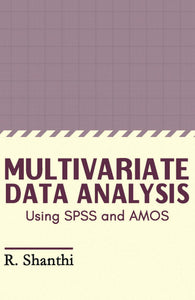# MULTIVARIATE DATA ANALYSIS : Using SPSS and AMOS

Vendor

MJP PUBLISHERS

Type

Books

Categories
200 in stock.
Quantity must be 1 or more
Regular price Rs. 1,595.00

Multivariate Data Analysis Introduction to SPSS Outliers Normality Test of Linearity Data Transformation Bootstrapping Homoscedasticity Introduction to IBM SPSS – AMOS Multivariate Analysis of Variance (MANOVA) One Way Manova in SPSS Multiple Regression Analysis Binary Logistic Regression Factor Analysis Exploratory Factor Analysis Confirmatory Factor Analysis Cluster Analysis K - Mean Cluster Analysis Hierarchical Cluster Analysis Discriminant Analysis Correspondence Analysis Multidimensional Scaling Example - Multidimensional Scaling (ALSCAL) Neural Network Decision Trees Path Analysis Structural Equation Modeling Canonical Correlation
Sale

Unavailable

Sold Out